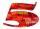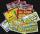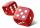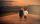# Prime number

Jan wrote any number from 1 to 20. What is the probability that he wrote the prime number?

Result

p =  0.4

#### Solution:Leave us a comment of example and its solution (i.e. if it is still somewhat unclear...):

Showing 0 comments:Be the first to comment!#### To solve this example are needed these knowledge from mathematics:

Our percentage calculator will help you quickly calculate various typical tasks with percentages. Would you like to compute count of combinations?

## Next similar examples:

1. NumbersWrite smallest three-digit number, which in division 5 and 7 gives the rest 2.
2. PercentsHow many percents is 900 greater than the number 750?
3. Jane classWhen asked how many students are in class, Jane said, if we increase the number of students in our class by hundred % and then add half the number of students, we get 100. How many students are in Jane's class?
4. PercentCalculate how many % is the number 26.25 less than the number 105.
5. Divisible by 5How many three-digit odd numbers divisible by 5, which are in place ten's number 3?
6. Probability - ticketsWhat is the probability that when you have 25 tickets in 5000 that you wins the first (one) prize?
7. Sum 10What is the probability that two dice throw twice in a row will result the sum of 10?
8. Percentages52 is what percent of 93?
9. BasketballPeter and Franta threw to the basket. Each had 20 attempts. Peter scored thirteen and twelve Franta. Give them success in percentage.
10. Highway repairThe highway repair was planned for 15 days. However, it was reduced by 30%. How many days did the repair of the highway last?
11. Frameworks is badCalculate how many percent will increase the length of an HTML document, if any ASCII character unnecessarily encoded as hexadecimal HTML entity composed of six characters (ampersand, grid #, x, two hex digits and the semicolon). Ie. space as: &#x20;
12. The ballThe ball was discounted by 10 percent and then again by 30 percent. How many percent of the original price is now?
13. PersonsPersons surveyed:100 with result: Volleyball=15% Baseball=9% Sepak Takraw=8% Pingpong=8% Basketball=60% Find the average how many like Basketball and Volleyball. Please show your solution.
14. Profit gainIf 5% more is gained by selling an article for Rs. 350 than by selling it for Rs. 340, the cost of the article is:
15. Cinema ticketsCinema sold 180 tickets this Thursday, which is 20%. Monday 14%, Tuesday 6%, Wednesday 9%, Friday 24%, Saturday 12%, and Sunday 15%. How many tickets were sold per week?
16. Test pointsIf you earned 80% of the possible 40 points, how many points did you miss to get 100%?
17. GirlsThe children's competition was attended by 63 girls, which is 30% of all children's participants. How many children attended this competition?